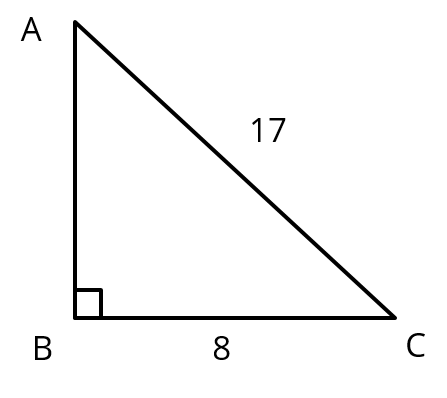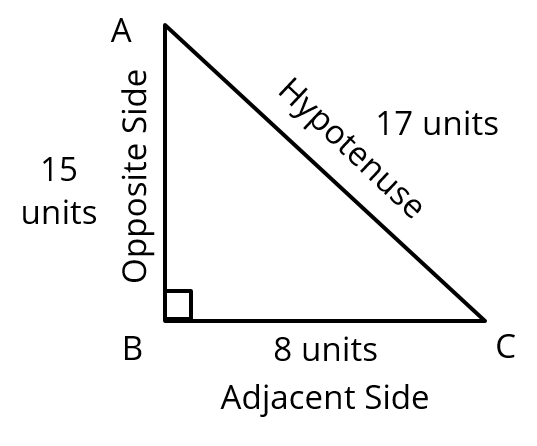# Extra Questions For Class 10 Maths Chapter 8 | Q1

#### Trigonometry | Trigonometric Ratios & Pythagoras Theorem

This CBSE class 10 Maths practice question is from the topic Trigonometry. It tests your understanding of the concepts of trigonometric ratios of a right triangle and application of Pythagoras Theorem. This extra question also helps you remember a popular Pythagorean Triplet.

Question 1 : Consider right triangle ABC, right angled at B. If AC = 17 units and BC = 8 units determine all the trigonometric ratios of angle C.## NCERT Solution to Class 10 Maths

### Explanatory Answer | Trigonometry Important Questions Q1

#### Given Data

Length of the side BC = 8 units
Length of the side AC = 17 units#### Step 1: Calculate the length of AB

In Δ ABC, using Pythagoras theorem, AB = $\sqrt{AC^2− BC^2 }$= $\sqrt{17^2− 8^2}$
$\sqrt{289−64}$ = $\sqrt{225}$
AB = 15 units

#### Step 2: Calculate the trigonometric ratios of angle C

sin C = $\frac{\text{opposite side}}{\text{hypotenuse}}$=$\frac{\text{AB}}{\text{AC}}$= $\frac{15}{17}$
cos C = $\frac{\text{adjcent side}}{\text{hypotenuse}}$=$\frac{\text{BC}}{\text{AC}}$= $\frac{8}{17}$
tan C =$\frac{\text{opposite side}}{\text{adjcent side}}$ =$\frac{\text{AB}}{\text{BC}}$= $\frac{15}{8}$
cot C =$\frac{\text{1}}{\text{tan ⁡C}}$ =$\frac{\text{adjcent side}}{\text{opposite side}}$ = $\frac{\text{BC}}{\text{AB}}$ = $\frac{8}{15}$
sec C = $\frac{\text{1}}{\text{cos ⁡C}}$ = $\frac{\text{hypotenuse}}{\text{adjcent side}}$ = $\frac{\text{AC}}{\text{BC}}$= $\frac{17}{8}$
cosec C = $\frac{\text{1}}{\text{sin ⁡C}}$ = $\frac{\text{hypotenuse}}{\text{opposite side}}$ =$\frac{\text{AC}}{\text{AB}}$= $\frac{17}{15}$

#### Try CBSE Online CoachingClass 10 Maths

Register in 2 easy steps and
Start learning in 5 minutes!